# Bhāskara II

Bhaskara (1114 – 1185), also known as Bhaskara II and Bhaskara Achārya ("Bhaskara the teacher"), was an Indian mathematician and astronomer. He became head of the astronomical observatory at Ujjain, continuing the mathematical tradition of Varahamihira and Brahmagupta.

## Contents

In many ways, Bhaskara represents the peak of mathematical and astronomical knowledge in the twelfth century. He reached an understanding of calculus, astronomy, the number systems, and solving equations, which were not to be achieved anywhere else in the world for several centuries. His main works were the Lilavati (dealing with arithmetic), Bijaganita (Algebra) and Siddhanta Shiromani (written in 1150) which consists of two parts: Goladhyaya (sphere) and Grahaganita (mathematics of the planets).

## General

Bhaskara, born in 1114 C.E. (1114 – 1185), also known as Bhaskara II and Bhaskara Achārya ("Bhaskara the teacher"), was an Indian mathematician and astronomer. He was born near Bijjada Bida (in present day Bijapur district, Karnataka state, South India) into the Deshastha Brahmin family. Bhaskaracharya, as he was known in India, was born to Mahesvara, another famous astrologer. He became head of the astronomical observatory at Ujjain, the leading mathematical center in India at the time, thus continuing the mathematical tradition of previously renowned mathematicians, Varahamihira and Brahmagupta.

Bhaskaracharya learnt mathematics from his father. After being introduced to the works of a previous famous mathematician, Brahmagupta, Bhaskaracharya was so inspired that he devoted himself to mathematics for the rest of his life. After his daughter, Lilavati, was widowed at the age of six, he even influenced her to study mathematics—it is not known, however, how great of a mathematician she became. When it came to algebra, Bhaskaracharya followed Brahmagupta’s work closely as his guru, and went about extending Brahmagupta’s works.

As a mathematician, Bhaskara represents the peak of mathematical and astronomical knowledge in the twelfth century. As J. J. O’Connor and E. F. Robertson stated in their article for the School of Mathematics and Statistics, “[Bhaskaracharya] reached an understanding of the number systems and solving equations which was not to be achieved in Europe for several centuries.” Bhaskaracharya was the first mathematician to write a work with full and systematic use of the decimal number system. His main work, written when he was only 30, includes the Siddhanta Siroman (written in 1150), which was segmented into four—these were the Lilavati (dealing with arithmetic), Bijaganita (Algebra), “Goladhyaya” (sphere), and “Grahaganita” (mathematics of the planets). There are also “Karanakutuhala” or “Brahmatulya,” a simplified version of the “Siddhanta Shiromani” and the “Vivarana,” a commentary on the “Shishyadhividdhidatantra.”

The books written by Bhaskaracharya were essentially textbooks, and had been simplified to help and stimulate student’s interests. The book became so well-known that even four or five centuries after it was written, it was translated into Persian.

Bhaskaracharya was known as an original thinker. Dilip M. Salwi of Our Scientists stated, “He was the first mathematician to declare confidently that any term divided by zero is infinity and the sum of any term and infinity is infinity” (though we now know that any term divided by zero is undefined). Still, he has made several major contributions to the world of mathematics. He is the first to introduce Chakrawal, a cyclic method of solving algebraic equations. In fact, it took six centuries after his finding for European mathematicians such as Galois, Euler, and Lagrange to rediscover this method and call it “inverse cyclic” method of solution.

Any trace of calculus first appeared in Bhaskaracharya’s works—Salwi stated, “Determination of the area and volume of a sphere in a rough integral calculus manner was also mentioned for the first time in his book. It contained important formulas and theorems in trigonometry and permutation and combination.” Though unknown by most, Bhaskaracharya can be considered the founder of differential calculus, for it was he who founded such methods centuries before Isaac Newton and Gottfried Leibniz came about it. At his time, no one took note of his great achievements. In astronomy, Bhaskaracharya is renowned for his concept of Tatkalikagati, instantaneous motion in physical terms. This advance allows astronomers to accurately measure planetary movements.

He wrote his second book, “Karanakutuhala,” at the age of 69. This consists of various astronomical calculations and remains, to this day, a reference book in the making the calendars based on astronomical happenings (analogical to horoscopes and numerology based systems).

## Legends

Lilavati, his book on arithmetic, is the source of interesting legends that assert that it was written for his daughter, Lilavati. In one of these stories, found in a Persian translation of Lilavati, Bhaskaracharya studied Lilavati's horoscope and predicted that her husband would die soon after the marriage if the marriage did not take place at a particular time. To prevent that, he placed a cup with a small hole at the bottom of a vessel filled with water, arranged so that the cup would sink at the beginning of the propitious hour. He put the device in a room with a warning to Lilavati to not go near it. In her curiosity though, she went to look at the device and a pearl from her nose ring accidentally dropped into it, thus upsetting it. The marriage took place at wrong time and she was soon widowed.

## Mathematics

Some of Bhaskara's contributions to mathematics include the following:

• A proof of the Pythagorean theorem by calculating the same area in two different ways and then canceling out terms to get a2 + b2 = c2.
• In Lilavati, solutions of quadratic, cubic and quartic indeterminate equations.
• Solutions of indeterminate quadratic equations (of the type ax2 + b = y2).
• Integer solutions of linear and quadratic indeterminate equations (Kuttaka). The rules he gives are (in effect) the same as those given by the renaissance European mathematicians of the seventeenth century.
• A cyclic, Chakravala method for solving indeterminate equations of the form ax2 + bx + c = y. The solution to this equation was traditionally attributed to William Brouncker in 1657, though his method was more difficult than the chakravala method.
• His method for finding the solutions of the problem x2 − ny2 = 1 (so-called "Pell's equation") is of considerable interest and importance.
• Solutions of Diophantine equations of the second order, such as 61x2 + 1 = y2. This very equation was posed as a problem in 1657 by the French mathematician Pierre de Fermat, but its solution was unknown in Europe until the time of Euler in the eighteenth century.
• Solved quadratic equations with more than one unknown, and found negative and irrational solutions.
• Preliminary concept of mathematical analysis.
• Preliminary concept of infinitesimal calculus, along with notable contributions towards integral calculus.
• He conceived differential calculus, after discovering the derivative and differential coefficient.
• Stated Rolle's theorem, a special case of one of the most important theorems in analysis, the mean value theorem. Traces of the general mean value theorem are also found in his works.
• Calculated the derivatives of trigonometric functions and formulae. (See Calculus section below.)
• In Siddhanta Shiromani, Bhaskara developed spherical trigonometry along with a number of other trigonometrical results. (See Trigonometry section below.)

### Arithmetic

Bhaskara's arithmetic text Lilavati covers the topics of definitions, arithmetical terms, interest computation, arithmetical and geometrical progressions, plane geometry, solid geometry, the shadow of the gnomon, methods to solve indeterminate equations, and combinations.

Lilavati is divided into 13 chapters and covers many branches of mathematics, arithmetic, algebra, geometry, and a little trigonometry and mensuration. More specifically the contents include:

• Definitions.
• Properties of zero (including division, and rules of operations with zero).
• Further extensive numerical work, including use of negative numbers and surds.
• Estimation of π.
• Arithmetical terms, methods of multiplication, and squaring.
• Inverse rule of three, and rules of 3, 5, 7, 9, and 11.
• Problems involving interest and interest computation.
• Arithmetical and geometrical progressions.
• Plane geometry.
• Solid geometry.
• Permutations and combinations.
• Indeterminate equations (Kuttaka), integer solutions (first and second order). His contributions to this topic are particularly important, since the rules he gives are (in effect) the same as those given by the renaissance European mathematicians of the seventeenth century, yet his work was of the twelfth century. Bhaskara's method of solving was an improvement of the methods found in the work of Aryabhata and subsequent mathematicians.

His work is outstanding for its systemization, improved methods and the new topics that he has introduced. Furthermore the Lilavati contained excellent recreative problems and it is thought that Bhaskara's intention may have been that a student of 'Lilavati' should concern himself with the mechanical application of the method.

### Algebra

His Bijaganita ("Algebra") was a work in twelve chapters. It was the first text to recognize that a positive number has two square roots (a positive and negative square root). His work Bijaganita is effectively a treatise on algebra and contains the following topics:

• Positive and negative numbers.
• Zero.
• The 'unknown' (includes determining unknown quantities).
• Determining unknown quantities.
• Surds (includes evaluating surds).
• Kuttaka (for solving indeterminate equations and Diophantine equations).
• Simple equations (indeterminate of second, third and fourth degree).
• Simple equations with more than one unknown.
• Indeterminate quadratic equations (of the type ax2 + b = y2).
• Solutions of indeterminate equations of the second, third and fourth degree.
• Quadratic equations with more than one unknown.
• Operations with products of several unknowns.

Bhaskara derived a cyclic, chakravala method for solving indeterminate quadratic equations of the form ax2 + bx + c = y. Bhaskara's method for finding the solutions of the problem Nx2 + 1 = y2 (the so-called "Pell's equation") is of considerable importance.

He gave the general solutions of:

• Pell's equation using the chakravala method.
• The indeterminate quadratic equation using the chakravala method.

He also solved:

• Cubic equations.
• Quartic equations.
• Indeterminate cubic equations.
• Indeterminate quartic equations.
• Indeterminate higher-order polynomial equations.

### Trigonometry

The Siddhanta Shiromani (written in 1150) demonstrates Bhaskara's knowledge of trigonometry, including the sine table and relationships between different trigonometric functions. He also discovered spherical trigonometry, along with other interesting trigonometrical results. In particular Bhaskara seemed more interested in trigonometry for its own sake than his predecessors who saw it only as a tool for calculation. Among the many interesting results given by Bhaskara, discoveries first found in his works include the now well known results for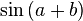$\sin\left(a + b\right)$ and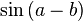$\sin\left(a - b\right)$:

•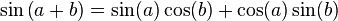$\sin\left(a + b\right) = \sin(a) \cos(b) + \cos(a) \sin(b)$
•$\sin\left(a - b\right) = \sin(a) \cos(b) - \cos(a) \sin(b)$

### Calculus

His work, the Siddhanta Shiromani, is an astronomical treatise and contains many theories not found in earlier works. Preliminary concepts of infinitesimal calculus and mathematical analysis, along with a number of results in trigonometry, differential calculus and integral calculus that are found in the work are of particular interest.

Evidence suggests Bhaskara was acquainted with some ideas of differential calculus. It seems, however, that he did not understand the utility of his researches, and thus historians of mathematics generally neglect his outstanding achievement. Bhaskara also goes deeper into the 'differential calculus' and suggests the differential coefficient vanishes at an extremum value of the function, indicating knowledge of the concept of 'infinitesimals'.

• There is evidence of an early form of Rolle's theorem in his work:
• If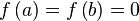$f\left(a\right) = f\left(b\right) = 0$ then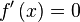$f'\left(x\right) = 0$ for some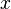$\ x$ with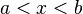$\ a < x < b$
• He gave the result that if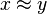$x \approx y$ then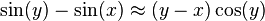$\sin(y) - \sin(x) \approx (y - x)\cos(y)$, thereby finding the derivative of sine, although he never developed the general concept of differentiation.
• Bhaskara uses this result to work out the position angle of the ecliptic, a quantity required for accurately predicting the time of an eclipse.
• In computing the instantaneous motion of a planet, the time interval between successive positions of the planets was no greater than a truti, or a fraction of a second, and his measure of velocity was expressed in this infinitesimal unit of time.
• He was aware that when a variable attains the maximum value, its differential vanishes.
• He also showed that when a planet is at its farthest from the earth, or at its closest, the equation of the center (measure of how far a planet is from the position in which it is predicted to be, by assuming it is to move uniformly) vanishes. He therefore concluded that for some intermediate position the differential of the equation of the center is equal to zero. In this result, there are traces of the general mean value theorem, one of the most important theorems in analysis, which today is usually derived from Rolle's theorem. The mean value theorem was later found by Parameshvara in the fifteenth century in the Lilavati Bhasya, a commentary on Bhaskara's Lilavati.

Madhava (1340-1425) and the Kerala School mathematicians (including Parameshvara) from the fourteenth century to the sixteenth century expanded on Bhaskara's work and further advanced the development of calculus in India.

## Astronomy

The study of astronomy in Bhaskara's works is based on the heliocentric solar system of gravitation earlier propunded by Aryabhata in 499, where the planets follow an elliptical orbit around the Sun, and the law of gravity described by Brahmagupta in the seventh century. Bhaskara's contributions to astronomy include accurate calculations of many astronomical results based on this heliocentric solar system of gravitation. One of these contributions is his accurate calculation of the sidereal year, the time taken for the Earth to orbit the Sun, as 365.2588 days. The modern accepted measurement is 365.2596 days, a difference of just one minute (analyzed by naked eyes and this accuracy is achieved in the absence of any sophisticated instrument).

His mathematical astronomy text Siddhanta Shiromani is written in two parts: the first part on mathematical astronomy and the second part on the sphere.

The twelve chapters of the first part cover topics such as:

• Mean longitudes of the planets.
• True longitudes of the planets.
• The three problems of diurnal rotation.
• Syzygies.
• Lunar eclipses.
• Solar eclipses.
• Latitudes of the planets.
• Risings and settings.
• The Moon's crescent.
• Conjunctions of the planets with each other.
• Conjunctions of the planets with the fixed stars.
• The patas of the Sun and Moon.

The second part contains thirteen chapters on the sphere. It covers topics such as:

• Praise of study of the sphere.
• Nature of the sphere.
• Cosmography and geography.
• Planetary mean motion.
• Eccentric epicyclic model of the planets.
• The armillary sphere.
• Spherical trigonometry.
• Ellipse calculations.
• First visibilities of the planets.
• Calculating the lunar crescent.
• Astronomical instruments.
• The seasons.
• Problems of astronomical calculations.

He also showed that when a planet is at its furthest from the Earth, or at its closest, the equation of the centre (measure of how far a planet is from the position it is to be predicted to be in by assuming it to movie uniformly) vanishes. He therefore concluded that for some intermediate position the differential of the equation of the centre is equal to zero.

## Legacy

Some scholars have suggested that Bhaskara's work influenced later developments in the Middle East and Europe. His work was perhaps known to Islamic mathematicians as soon as it was written, and influenced their subsequent writings. The results thus became indirectly known in Europe by the end of the twelfth century, but the text itself was not introduced until much later (Ball, 1960). (See Possible transmission of Kerala mathematics to Europe for other evidence.) The Mughal emperor Akbar commissioned a famous Persian translation of the Lilavati in 1587.

There have also been several allegedly unscrupulous attempts to argue that there are traces of Diophantine influence in Bhaskara's work, but this is seen as an attempt by Eurocentric scholars to claim European influence on many great non-European works of mathematics. Particularly in the field of algebra, Diophantus only looked at specific cases and did not achieve the general methods of the Indians. The study of Diophantine equations in India can also be traced back to the Sulba Sutras written from 800 B.C.E. to 500 B.C.E., which pre-date Diophantus' work by many centuries.

## Final Days

Bhaskara was a natural born teacher and mathematician. As is common at his time, generations of a family would be mathematicians, with each father passing on knowledge to their sons. Bhaskara himself passed on his knowledge to his son, Loksamudra. Bhaskaracharya passed away in 1185 C.E. at Ujjain.